Author: Oscar Cronquist Article last updated on April 19, 2018

The Mod function returns the remainder after a number is divided by divisor. The Mod function is short for the Modulo operation (link to wikipedia).

MOD(number, divisor)

• Number - The number for which you want to find the remainder.
• Divisor - The number by which you want to divide number.

### What does it mean - finds remainder after a number is divided by divisor?

The remainder is what is left after a division. If you divide 15 with 2 you get 7 and 1 is left over. 2*7 equals 14 and 15 minus 14 equals 1. 1 is the remainder or is left over. MOD(15,2) = 1. Sometimes nothing is left over, like 6 divided by 3 equals 2. The remainder is 0.

MOD(number, divisor) can be expressed like this: number - divisor*INT(number/divisor)

Example 1,

11 divided by 3 returns 2.

number - divisor*INT(number/divisor)

11-3*INT(11/3)

becomes

11-3*3

becomes

11-9 equals 2.

Example 2,

Here is a different approach.

14 divided by 5 is 2 and 4/5

Take the remainder (4/5) and multiply it with the divisor (5)

5* (4/5) equals 4. 4 is the answer.

### Many more examples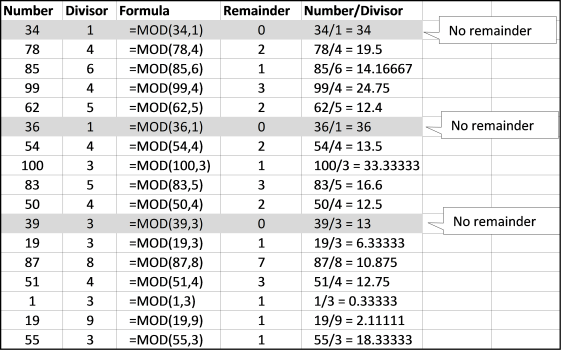### Practical examples

Apply a formula every n-th row

The following formula concatenates cells i column A every third row:

=IF(MOD(ROW(), 3)=0, CONCATENATE(A1, A2, A3), "")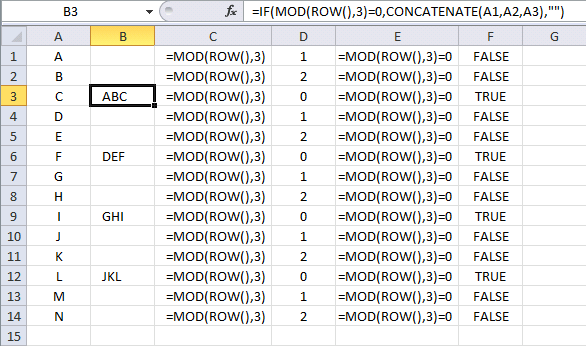Lets begin with ROW() in cell B3. It is dynamic and changes for each row. It returns the current row number, example in cell B3 ROW() returns 3.

Then the MOD function takes 3 and divides it with 3. MOD(ROW(), 3) returns 0.  The remainder is zero in cell B3. You can see this part of the formula in column C and the result in column D.

MOD(ROW(),3)=0 is logical expression, it checks if the output from the MOD function is equal to 0. In every third row it is and MOD(ROW(),3)=0 returns TRUE. You can see this part of the formula in column E and the result in column F.

The IF function returns CONCATENATE(A1, A2, A3) if logical expression is TRUE or a blank if logical expression is FALSE.

There are relative cell references in the CONCATENATE function and they change in each cell. Don´t know much about relative and absolute cell references? Read this: Absolute and relative references in excel

=IF(MOD(ROW(), 3)=0, CONCATENATE(A1, A2, A3), "")

Highlight every n-th row

You can use the same technique to highlight every second row with conditional formatting.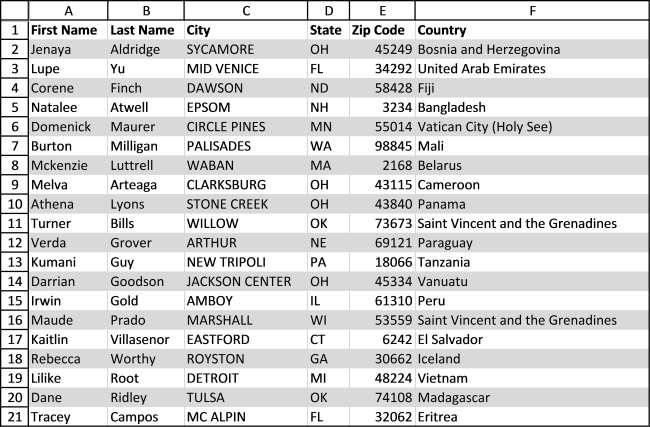Here is how to apply conditional formatting to a cell range. Select a cell range. Go to the "Home" tab on the ribbon. Click Conditional formatting button. Click "New Rule...". Click "Use a formula to determine which cells to format:".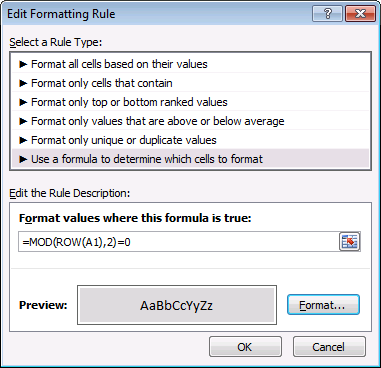Type the formula below. Click the "Format" button. Go to tab "Fill". Pick a color. Click OK twice.

Conditional formatting formula: =MOD(ROW(A1),2)=0

If you want to highlight every third row change the formula to =MOD(ROW(A1),3)=0.

Highlight every other group

Example 1,

This conditional formatting rule highlights every other group based on values in column A.Conditional formatting formula: =MOD(SUM(1/COUNTIF(\$A\$2:\$A2,\$A\$2:\$A2)),2)

Column A must be sorted.

Example 2, groups based on values in column A, B and C.Conditional formatting formula:

=MOD(SUM(1/COUNTIFS(\$A\$2:\$A2, \$A\$2:\$A2, \$B\$2:\$B2, \$B\$2:\$B2, \$C\$2:\$C2, \$C\$2:\$C2)), 2)

Make sure you get the relative and absolute cell references right.

Mod function returns the fractional part

If you use 1 as divisor the mod function returns the fraction of a number. number - INT(number/divisor)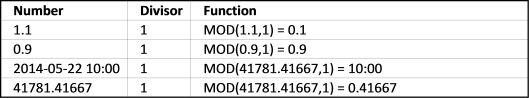You can use this to return only hours from a cell containing date and time, see row 3 in the picture above.

QUOTIENT function returns the INTEGER portion of a division

Quotient function returns a number without the fractional component.

78 / 4 = 19.5

The quotient is 19. Here are more examples, the quotients are in column C.QUOTIENT(numerator, denominator) uses the same arguments as the MOD function.

C Pearson extracts vectors from a matrix with the MOD function

Extracting Vectors From A Matrix

### MOD function won´t work with really large numbers

There seems to be a problem with large numbers and Microsoft knows about it: http://support.microsoft.com/kb/119083

The MOD() function returns the #NUM! error if the following condition is true:
('divisor' * 134217728) is less than or equal to 'number'

### Download excel *.xlsx file

You can find all examples in this post in the file below.

Mod function.xlsx

If you want to learn more about array formulas join Advanced excel course.